# T Test for Dependent Groups Solution

### Example homework problem:

Twelve people completed a difficult hand-eye coordination task twice: once in a quiet room, and once in the same room while loud rock-and-roll music was being played. The order in which subjects experienced these conditions was counterbalanced: half performed in the quiet environment first, half performed in the loud environment first.

The researcher measured the number of errors committed by the participant while  performing. Thus, the lower the score, the better the performance. Here are the results:

 Participant: 1 2 3 4 5 6 7 8 9 10 11 12 Quiet Environment: 14 21 24 16 18 20 15 20 17 16 19 12 Loud Environment: 21 22 21 19 18 25 17 18 21 24 21 15

Was there a significant difference in performance between the two treatment conditions  (alpha = .05)?

The above problem involves an experiment with two treatment conditions. The goal of your
test is to see if there is significant mean difference between the two treatment conditions.

We will test for a significant mean difference between the treatment conditions with a t-test for related samples: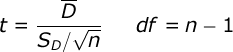This t test is really simple — t is equal to the mean of the difference scores divided by the standard error of the difference scores. You will see how to get the difference scores below. n equals the number of participants, or the number of pairs of scores.

Compute test statistic. First, compute a difference score for each subject: i.e., for each subject, subtract their score in the loud environment from their score in the quiet environment. Here are your difference scores:

 Participant: 1 2 3 4 5 6 7 8 9 10 11 12 Quiet Environment: 14 21 24 16 18 20 15 20 17 16 19 12 Loud Environment: 21 22 21 19 18 25 17 18 21 24 21 15 Difference Score: -7 -1 +3 -3 0 -5 -2 +2 -4 -8 -2 -3

Now, your analysis will involve only these difference scores. Compute the mean and the standard deviation of these difference scores. In our study, ΣD = -30, ΣD² = 194, and n = 12. So, the mean of the difference scores is equal to -2.50, and the standard deviation of the difference scores is equal to 3.29. If you would like help computing these descriptive statistics, read the documentation for the explore procedure. Compute these statistics for yourself now. When you compute t, you will find that: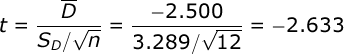Conduct hypothesis test. In our case, n = 12 so the df for our t test will be 11. Let us assume that we will employ a non-directional (i.e., two-tailed) test with alpha = .05. From your table of critical values for the t test, you will find that if the absolute value of our obtained t is greater in value than 2.201, we would conclude that the noise level in the environment had a significant effect on performance.

Since 2.633 is greater than 2.201, we would conclude that the participants’ performance was significantly affected by the noise level in the environment.

Effect size. To describe the effect size for this study, we will first compute r²: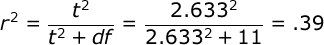r² is an estimate of the proportion of variance in our dependent variable that can be accounted for by our manipulation of the independent variable. So, we could conclude that 19% of the variance in recall was due to our manipulation of the encoding instructions.

However, many researchers prefer to use an unbiased effect size estimate — one that takes into account the fact that we are doing small sample research. So, we will also compute  Omega Squared: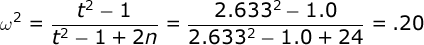We would conclude that our manipulation of encoding instructions accounted for 14% of the variance in memory performance.

Finally, we will compute Cohen’s D: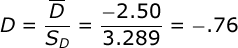which is simply the mean of the difference scores divided by the standard deviation of the difference scores. What Cohen’s D tells us is that there is .76 of a standard deviation difference between our groups.